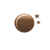This chart combines two scatter charts, where the first and second chart's data is retrieved from the same file.

Data for the second scatter chart is built by rchart's built in regression calculation. This function does a basic regression analysis and provides the caculated formula and data of this analysis. Together with the regression formula also the calculated coefficient of determination as "R2" is displayed. The coefficient of determination is the proportion of variability in the data that is accounted for by the calculated formula and it will range from "0" (no correlation) to "1" (perfect correlation).

To combine these two scatter charts, parameter "combine_chart" is set to "true". This causes that second chart is drawn into the same canvas as first chart as both charts use the same id ("cvs"). It is important that both charts have the same scale (see "width", "height", "xmax", "ymax", "xmin" and "ymin").

#### Parameter for creation of chart 1.

```{rgraph type="Scatter" width="600" height="400" data="file" file="plugins/content/rgraph/doc/scatterdata.json"title="Body Size versus Length of Arms" marginLeft="80" backgroundGridAutofit="false" xaxisScaleMin="150"xaxisScaleMin="150" xaxisScaleMax="203" yaxisScaleMin="150" yaxisScaleMax="200" yaxisScaleUnitsPost=" cm"yaxisTitle="Body Size" xaxisTitle="Length of Arms in cm" x_step="2" yaxisTitlePos="0.30"}cvs{/rgraph}
```

#### Parameter for creation of chart 2.

```{rgraph type="Scatter" width="600" height="400" data="file" file="plugins/content/rgraph/doc/scatterdata.json" marginLeft="80" combine_chart="1"  xaxisScaleMin="150" xaxisScaleMax="203" yaxisScaleMin="150" yaxisScaleMax="200" x_step="2" yaxisTickmarks="false"  calculate_regression="1" line="true" show_regression_formula="1"  keyPositionY="175" linewidth="2" xaxis="false" yaxis="false" xaxis="0" xaxisLabels="" yaxisLabelsCount="0" backgroundGrid="false" tickmarksStyle="null" scaleDecimals="2"  xmax="203" ymin="150"  ymax="200"}cvs{/rgraph}
```

[No canvas support]>

Important: If you want to test this sample via copy/paste, ensure that you paste with option "paste as plain text" otherwise imbedded html-codes may invalidate your input.
go back...

### Visitors

Today: 2
Yesterday: 56
This Week: 241
Last Week: 339
This Month: 379
Last Month: 1,626
Total: 231,811
023181130.3% Germany23.4% USA3.3% France2.8% Italy2.1% Indonesia2.0% Poland1.8% Netherlands1.8% Spain1.8% India

Total:

208

Countries

### Weather in Ashburn

9th December, 2023 - 9:47
Clear Sky37°F 31°F min 43°F max13:1722:47
Humidity: 87 %
Wind: 0.0 mph North
Pressure: 1,021 hPa
Visibility: 32,808 ft